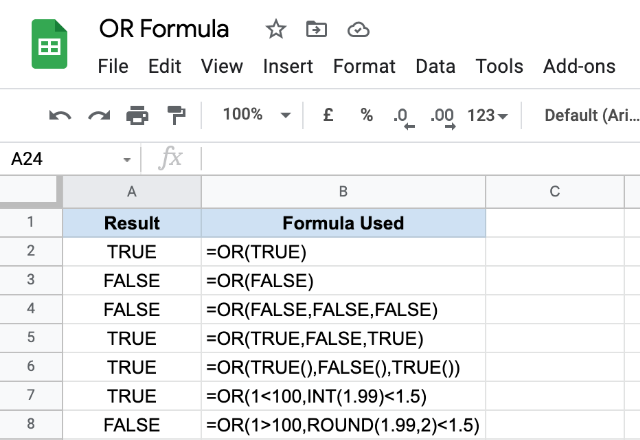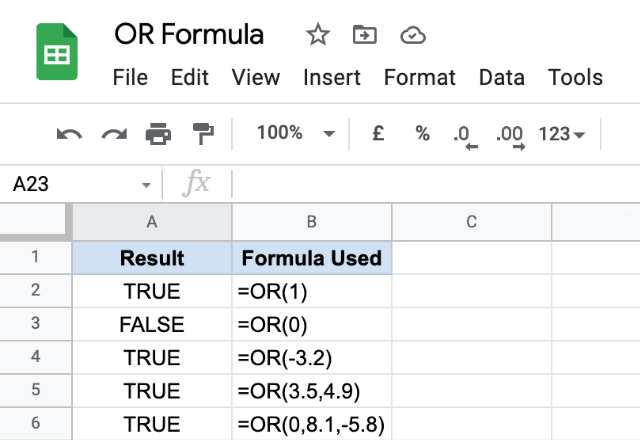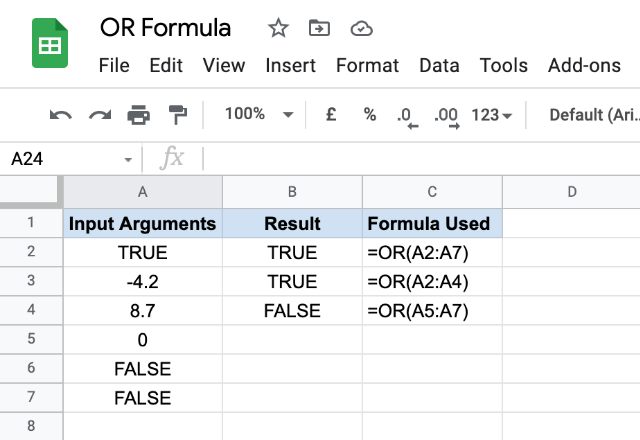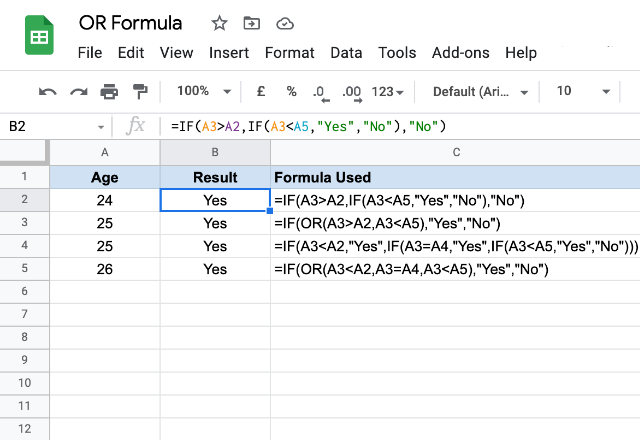# How to use the OR formula in Google SheetsThe OR formula in Google Sheets accepts logical expressions as input arguments, evaluates them to either a logical TRUE or FALSE. If all of the input arguments evaluate to FALSE, then the formula returns FALSE as the end result. However, if any of the input arguments evaluate to TRUE, the formula returns TRUE as an output.

### Syntax

OR(logical_expression1, [logical_expression2, …])

logical_expression1 – is an expression that evaluates to a logical TRUE or FALSE. This can be a direct expression or a reference to the cell that represents a logical expression.

[logical_expression2, …] – these are optional and additional expressions that return a logical TRUE or FALSE.

### Usage: OR Formula in Google Sheets

Let us try various combinations of input arguments within the OR formula and examine the results. You may have already guessed that the first two variations of the formula with a single input argument, aren’t that useful to us.It is interesting to note that the OR formula works even when we provide numbers as the input arguments. What happens when the Google Sheets application encounters a number when it is expecting a logical TRUE or FALSE? It simply converts them to a logical TRUE or FALSE. A zero is converted to FALSE and a non-zero to TRUE. Doesn’t really matter if the numbers contain decimals. Please see the examples in the snapshot below.The OR formula also takes a range of cells as an input argument, as illustrated in the image below.While it is hard to deny the utility of the OR formula in the real world, probably it has a limited application as a standalone formula. However, when it is used in conjunction with other formulas like IF, we can see its magic coming to life. In the snapshot below, consider the first and third formulas. The second and fourth formulas are their alternatives. Notice how simple it gets when we use OR instead of multi-level IF formula nesting.### OR formula

And there you go! Use the OR formula in Google Sheets to evaluate logical expressions.

If you’d like to learn more about the various formulas of Google Sheets, why not take a look at our blog post on the

Alternatively, check out related blog posts below!